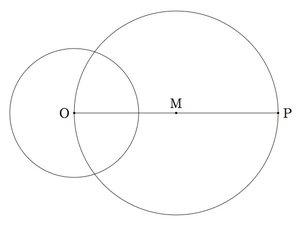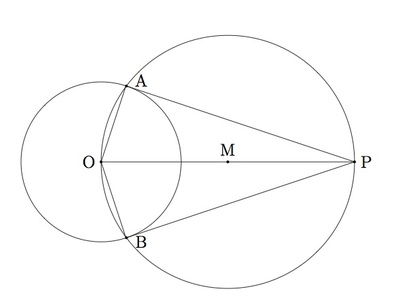# Tangent to a circle from a point

Alignments to Content Standards: G-C.A.4

Suppose $C$ is a circle with center $O$ and $P$ is a point ourside of $C$. Let $M$ be the midpoint of segment $\overline{OP}$ and let $D$ be the circle with center $M$ passing through $O$.Let $A$ and $B$ be the two points of intersection of $C$ and $D$, pictured below along with several line segments of interest:1. Show that angles $OAP$ and $OBP$ are right angles.
2. Show that $\overleftrightarrow{PA}$ and $\overleftrightarrow{PB}$ are tangent lines from $P$ to the circle $C$.

## IM Commentary

The construction of the tangent line to a circle from a point outside of the circle requires knowledge of a couple of facts about circles and triangles. First, students must know, for part (a), that a triangle inscribed in a circle with one side a diameter is a right triangle. This material is presented in the tasks''Right triangles inscribed in circles I.'' For part (b) students must know that the tangent line to a circle at a point is characterized by meeting the radius of the circle at that point in a right angle: more about this can be found in ''Tangent lines and the radius of a circle.''

Because of all of the prerequisites this is an ideal task for using some of these other constructions and it serves to reinforce their importance. It might be appropriate to study this problem in order to motivate the constructions required in the solution. Then students can return to this afterward as a reward for having mastered those techniques.

While the solution to this problem appears to be very short this is misleading as each step requires careful attention. One of appealing aspects of geometry and mathematics in general is the way results build upon one one another. It is vital that students see some of the more creative and sophisticated constructions like the one performed here in order to feel motivated to learn the supporting structures which make this possible.

If the distance from $P$ to $O$ is equal to $r$ then $P$ lies on the circle and so the tangent line from $P$ to $C$ is the tangent line of $C$ at $P$. If the distance from $P$ to $O$ is less than $C$ then $P$ lies inside $C$ and there is no tangent line from $P$ to $C$.

## Solution

Below is a picture of the different points and triangles used in the solution of the problem:1. Segment $\overline{OP}$ is a diameter of circle $D$ since the center of $D$ is the midpoint $M$ of this segment. The points $A$ and $B$ are also both on $D$ since they are the points of intersection of $C$ and $D$. The angles $OAP$ and $OBP$ are both right angles because $\overline{OP}$ is a diameter of circle $D$ and $A$ and $B$ are points on $D$: this means that triangles $OAP$ and $OBP$ are inscribed in circle $D$ and so the angle opposite the diameter must be a right angle.
2. Since angles $OAP$ and $OBP$ are right angles it follows that $\overleftrightarrow{PA}$ meets the radius $\overline{OA}$ in a right angle and similarly $\overleftrightarrow{PB}$ meets radius $\overline{OB}$ in a right angle. This means that $\overleftrightarrow{PA}$ is tangent to $C$ at $A$ and $\overleftrightarrow{PB}$ is tangent to $C$ at $B$.

Below is a picture with the two tangent lines constructed above: